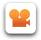Enter the content which will be displayed in sticky bar

# EventThe Engineering Value of the Cosmological Constant

Date: 2011-02-26 Time: 07:00 - 09:00 US/Pacific (1 decade 5 months ago)
America/Los Angeles: 2011-02-26 07:00 (DST)
America/New York: 2011-02-26 10:00 (DST)
America/Sao Paulo: 2011-02-26 11:00
Europe/London: 2011-02-26 14:00
Asia/Colombo: 2011-02-26 19:30
Australia/Sydney: 2011-02-27 01:00 (DST)

Where: Online Video ConferenceRecording Playback
This video conference used DimDim, now a private company.
The meeting can be replayed by clicking this link:
watch the meeting recording

Description

The basis of the new alternative energy knowledge is the realization that Einstein did not emphasize the physical properties of the vacuum and caused the cosmologists to assume that the cosmological constant, which is the energy density of interstellar space, is very small, while the quantum theorists calculated it to be enormously high. In reality, the engineering value of it is somewhere in the middle of both extreme values and could be surprisingly close to the old classical value of the energy density of the eighteenth century space fluid, called the ether. Charles Muses quoted the classical value to be about 1.4x10^30 Joules per cubic meter. Another value of 4.14x10^30 J/m^3 was calculated by Robert E.Var and published in Foundations of Physics, Vol. 5, No. 3, September 1975 in his paper "On a Mathematical Framework for Fundamental Theoretical Physics". Since 1 J/m^3 = .000145 psi, the above energy densities equal 0.6x10^26 psi and 2.03x10^26 psi respectively.

If the speed of light, by virtue of the analogy of the Lorentz factor [1-(v/c)^2]^.5 and the air shockwave equations term (1-kM^2)^.5, is treated like the speed of sound in air, then some interesting deductions follow. In air the velocity of sound squared equals the medium pressure or its energy density, divided by its mass density. Clark Maxwell, the 19th century scientist and the originator of the electrodynamic Maxwell equations, derived the equation c^2=1/epsilon mu, one and a half century ago, thereby fixing the interdependency of the three properties of non-matter space that we have known now for some time; namely the speed of ligfht, the electric permitivity of space and the magnettic susceptibility of space. Robert Var takes 1/epsilon as the energy density of space. His analysis allowed him to calculate the mass density of the ether to be 7.57 kg/cc. Not to bad a number for a medium that occupies more intervening space than the seperated atoms of matter.

The energy density of the cosmological constant has the same dimensionality as hydrostatic pressure [lbf inch / cubic inch] if it is divided by [inch] in the numerator as well as in the denominator, to give psi [lbf / square inch]. Herein lies the understanding of how forces come about: They are energy density gradients, field energy gradients, or changes in energy density arising from medium flow velocities in accordance with the fluid dynamic equations of Bernouilli, giving rise to negative pressure differentials, with respect to the ambient pressure for the medium at rest, in a vector direction, which then, while acting on a finite perpendicular surface area of X inch square, allow the removal of the [square inch] dimensionality of psi and then leaves nothing else than just the force [lbf]. Forces are thus energy density gradients due to steady and accelerating motion in energetic media. The media can be material, such as air, or non material, such as the vacuum medium.